﻿ Some New Generalizations of Fuzzy Average Code Word Length and their Bonds

### Some New Generalizations of Fuzzy Average Code Word Length and their Bonds

M.A.K. Baig, Mohd Afzal Bhat, Mohd Javid DarOPEN ACCESSPEER-REVIEWED

## Some New Generalizations of Fuzzy Average Code Word Length and their Bonds

M.A.K. Baig1,, Mohd Afzal Bhat1, Mohd Javid Dar1

1University of Kashmir, Hazratbal, Srinagar, India

### Abstract

In this communication, we propose a new generalizations of fuzzy average codeword length La and study its particular cases. The results obtained not only generalize the existing fuzzy average code word length but all the known results are the particular cases of the proposed length. Some new fuzzy coding theorems have also been proved.

• Baig, M.A.K., Mohd Afzal Bhat, and Mohd Javid Dar. "Some New Generalizations of Fuzzy Average Code Word Length and their Bonds." American Journal of Applied Mathematics and Statistics 2.2 (2014): 73-76.
• Baig, M. , Bhat, M. A. , & Dar, M. J. (2014). Some New Generalizations of Fuzzy Average Code Word Length and their Bonds. American Journal of Applied Mathematics and Statistics, 2(2), 73-76.
• Baig, M.A.K., Mohd Afzal Bhat, and Mohd Javid Dar. "Some New Generalizations of Fuzzy Average Code Word Length and their Bonds." American Journal of Applied Mathematics and Statistics 2, no. 2 (2014): 73-76.

 Import into BibTeX Import into EndNote Import into RefMan Import into RefWorks

### 1. Introduction

Fuzzy sets play a significant role in many deployed systems because of their capability to model non-statistical imprecision. Consequently, characterization and quantification of fuzziness are important issues that affect the management of uncertainty in many system models and designs. Zadeh  introduced the concept of fuzzy sets in which imprecise knowledge can be used to define an event. A fuzzy set ‘A’ is represented as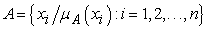where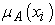gives the degree of belongingness of the element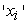to the set ‘A’. If every element of the set ‘A’ is ‘0’ or ‘1’, there is no uncertainty about it and a set is said to be crisp set. On the other hand, a fuzzy set ‘A’ is defined by a characteristic function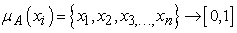.

The functionassociates with each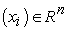grade of membership function. The importance of fuzzy set comes from the fact it can deal with imprecise and inexact information. Its application areas span from design of fuzzy controller to robotics and artificial intelligence. Many fuzzy measures have been discussed and derived by Kapur , Lowen , Pal and Bezdek  etc.

The basic noiseless coding theorems [6, 11] give the lower bound for the mean codeword length of a uniquely decipherable code in terms of Shannon’s  measure of entropy. Kapur  has established relationship between probabilistic entropy and coding. But, there are situations where probabilistic measure of entropy does not work. To tackle such situations, instead of taking the probability, the idea of fuzziness can be explored.

De Luca and Termini  introduced a measure of fuzzy entropy corresponding to Shannon’s  information theoretic entropy and is given by(1.1)

Bhandari and Pal  surveyed the literature on information measures of fuzzy sets and also gave some new measures. Thus corresponding to Renyi’s  entropy of order they suggested that the amount of ambiguity or fuzziness of order should be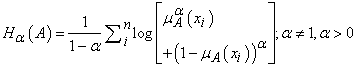(1.2)

Kapur  has taken measure of fuzzy entropy corresponding to Havrada Charvat  as(1.3)

Corresponding to Cambell’s  measure of entropy, the fuzzy entropy can be taken as(1.4)

Corresponding to Sharma and Taneja  measure of entropy of degree (,), Kapur  has taken the following measure of entropy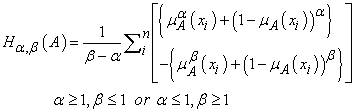(1.5)

Corresponding to Kapur  measure of entropy of degree (,), Kapur  has given measure of entropy for fuzzy sets as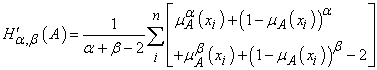(1.6)

Shannon  established the first noiseless coding theorem which states that for all uniquely decipherable codes, the lower bound for the arithmetic mean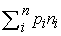lies between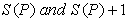, where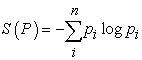is Shannon’s measure of entropy.

Mathai, A.M.  has given the measure of entropy as(1.7)

Corresponding to this measure, we propose the following average codeword length as:(1.8)

Corresponding to equation (1.7) we propose the following measure of fuzzy entropy as(1.9)

And the corresponding average codeword length as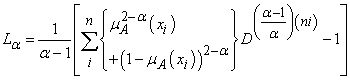(1.10)

Remark :

(I): When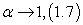tends to Shannon’s entropy given as(1.11)

(II): When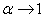(1.8) tends to average codeword length given by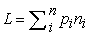(1.12)

In section 2, some noiseless coding theorems connected with fuzzy entropy corresponding to Mathai’s  entropy have been proved.

### 2. Fuzzy Noiseless Coding Theorems

Theorem2.1: For all uniquely decipherable codes(2.1)

Where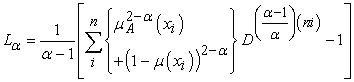Proof: By Holders inequality, we have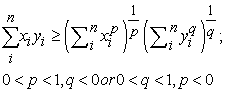(2.2)

Set;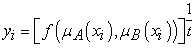and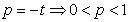,.

Thus equation (2.2) becomes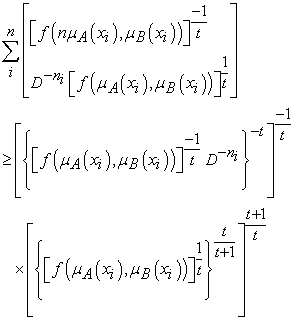Using Kraft’s inequality, we have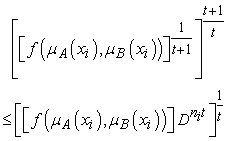or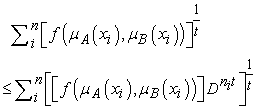or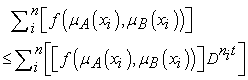(2.3)

Dividing both sides by t, we getSubtracting n from both sides, we get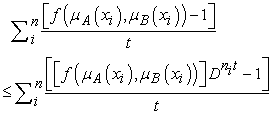(2.4)

Takingand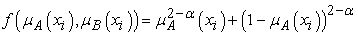Thus equation (2.4) becomes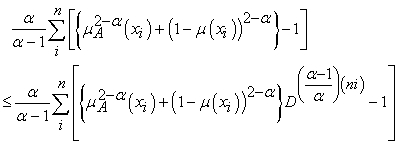Dividing both sides by α, we get(2.5)

That is.

Which proves the theorem.

Theorem 2.2: For all uniquely decipherable codes,(2.6)

Where,(2.7)

AndProof: Since from (2.5), we have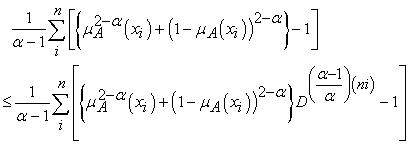Multiplying both sides by, we have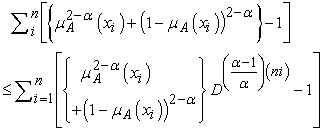(2.8)

Changing α to β, we have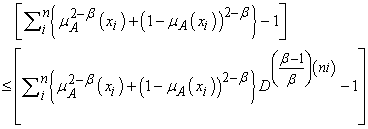(2.9)

Subtract (2.9) to (2.8), and divide by, we get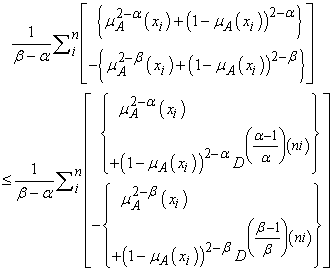(2.10)

That is. This proves the theorem.

Theorem 2.3: For all uniquely decipherable codes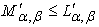(2.11)

Where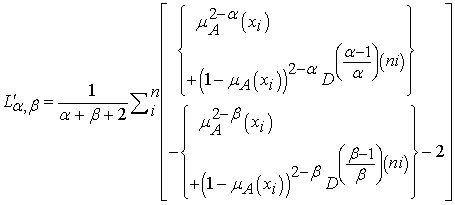(2.12)

Proof: The result can be easily proved by adding (2.8) and (2.9) and then dividing byTheorem 2.4: For all uniquely decipherable codes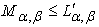(2.13)

Where(2.14)

And(2.15)

To prove this theorem, we first prove the following lemma.

Lemma 1: For all uniquely decipherable codes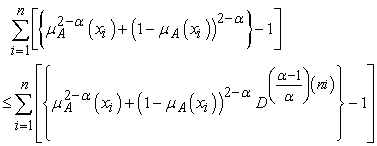Proof of the Lemma. From equation (2.3), we haveSubtracting ‘n’ from both sides, we getTaking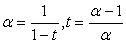, and, we have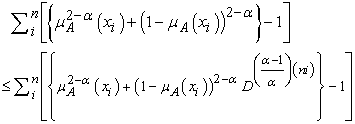(2.16)

Which proves the lemma

Proof of the theorem 2. 4

Changing α to β in (2.16), we have(2.17)

Dividing (2.17) to (2.16), we get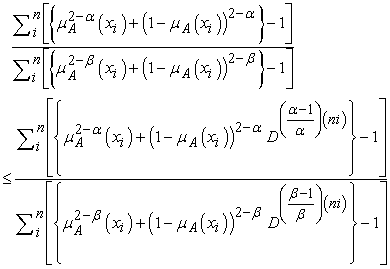Dividing both sides by β − α, we have(2.18). The R.H.S. is a new exponentiated mean codeword length of order α and type β and is defined as### References

  Bhandari, N. R. Pal, Some new information measures for fuzzy sets, Information Sciences 1993; Vol. 67, No. 3: pp. 209-228.In article CrossRef  Campbell, L.L., A coding theorem and Renyi’s entropy, Information and Control 1965; Vol. 8: pp. 423-429.In article CrossRef  De Luca, S. Termini, A Definition of Non-probabilistic Entropy in the Setting of fuzzy sets theory, Information and Control 1972; Vol.20: pp.301-312.In article CrossRef  Havrada, J. H., Charvat, F., Quantification methods of classificatory processes, the concepts of structural α entropy, Kybernetika 1967; Vol.3: pp. 30-35.In article  J.N.Kapur, Measures of Fuzzy Information, Mathematical Science Trust Society, New Delhi; 1997.In article  Kapur, J. N., A generalization of Campbell’s noiseless coding theorem, Jour. Bihar Math, Society 1986; Vol.10: pp.1-10.In article  Kapur, J. N., Entropy and Coding, Mathematical Science Trust Society, New Delhi; 1998.In article  Lowen, R., Fuzzy Set Theory–Basic Concepts, Techniques and Bibliography, Kluwer Academic Publication. Applied Intelligence 1996; Vol. 31, No. 3: pp.283-291.In article  Mathai, A.M., Rathie, P.N., Basic Concept in Information Theory and Statistics. Wiley Eastern Limited, New Delhi; 1975.In article  Pal, Bezdek, Measuring Fuzzy Uncertainty, IEEE Trans. of fuzzy systems 1994; Vol. 2, No. 2: pp.107-118.In article CrossRef  Renyi, A., On measures of entropy and information. Proceedings 4th Berkeley Symposium on Mathematical Statistics and Probability 1961; Vol.1: pp.541-561.In article  Shannon, C. E., A mathematical theory of communication. Bell System Technical Journal 1948; Vol.27: pp.379-423, 623-659.In article CrossRef  Sharma, B.D., Taneja, I. J., Entropies of typeα, β and other generalized measures of information theory, Matrika 1975; Vol.22: pp. 205-215.In article CrossRef  Zadeh, L. A., Fuzzy Sets, Inform, and Control 1966; Vol.8: pp.94-102.In article